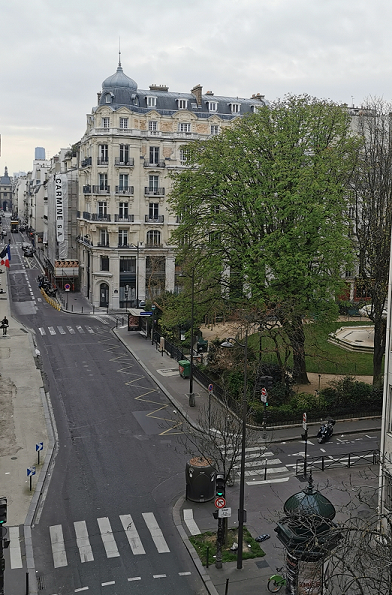# Lifting data… and washing machines: kernel computations from optical random features

Original article can be found here (source): Deep Learning on Medium## Throwing the random kitchen sinks at the problem

The major drawback of kernel methods is that given n data points, there is a need to compute the correlation for all pairs of points. If we write these correlations in a matrix form, we have an n×n kernel matrix that is really expensive to compute and store when the number of data points is large. Given current computational capabilities, exact kernel methods are therefore used only for average-sized datasets — n ～10⁴ points.

Let us assume a problem with n data points of dimension D. Using traditional kernel methods we would need to compute and store a matrix of elements. If n=1.000.000, this could require 1 TB of memory. Naively inverting this matrix to perform Kernel Ridge Regression  would cost n³ operations or about an exaop. As a result of this difficult scaling, several solutions have been proposed to overcome this limitation.

Ali Rahimi and Ben Recht developed the technique of random features [4, 5]: a distance-dependent kernel (such as the RBF) can be approximated in expectation by the dot product of randomized feature maps. The randomness is sampled from the Fourier transform of the kernel. For instance, for the RBF, we have: